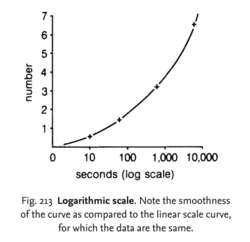# logarithmic scale

(redirected from Logarithmic Scales)
Also found in: Dictionary, Thesaurus, Financial, Encyclopedia.Fig. 213 Logarithmic scale . Note the smoothness of the curve as compared to the linear scale curve, for which the data are the same.

## logarithmic scale

a scale in which the values of a variable are expressed as logarithms. Such transformations of data are often employed to simplify the drawing of lines on a graph, where the variable values are spread over a wide range. See Fig. 213 . A good example of the use of log scales is seen in Fig. 182 where the LOG PHASE of a microbial GROWTH CURVE is illustrated. Compare LINEAR SCALE.
Collins Dictionary of Biology, 3rd ed. © W. G. Hale, V. A. Saunders, J. P. Margham 2005
References in periodicals archive ?
From these findings, the relationship between the infection manifested, in terms of the total area of the fruits, and the diagrammatic logarithmic scale which represents the percentage of damage the fungus caused in the fruit at different stages was developed.
As background to the log-log plots (Figure 3), Armitage and Doll (12,13) in 1954 demonstrated that the age-specific incidence rates of certain epithelial cancers have a linear slope when plotted against the age of diagnosis on logarithmic scales. This logarithmic transformation of population data confirmed a theoretical equation relating the sequential development of carcinoma to a series of molecular changes and cellular events, and thereby supporting the multistage process of carcinogenesis with a continuous increase in cancer rate as a function of age.
(The plot in figure 6 is on logarithmic scales, so the power-law dependence appears linear with slope [beta].)
The half-life of antibody to CHIKV was calculated by plotting the antibody titer versus age to 18 months on both linear and logarithmic scales. Using SPSS software (SPSS Inc., Chicago, IL, USA), we calculated the line of best fit by exponential regression (Figure 2).
Due to the characteristics of logarithmic scales, that means the geodetic measurements indicate an earthquake that was twice as big as the one shown by seismic measurements.

Site: Follow: Share:
Open / Close